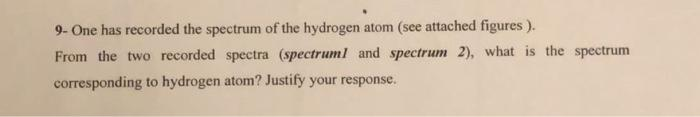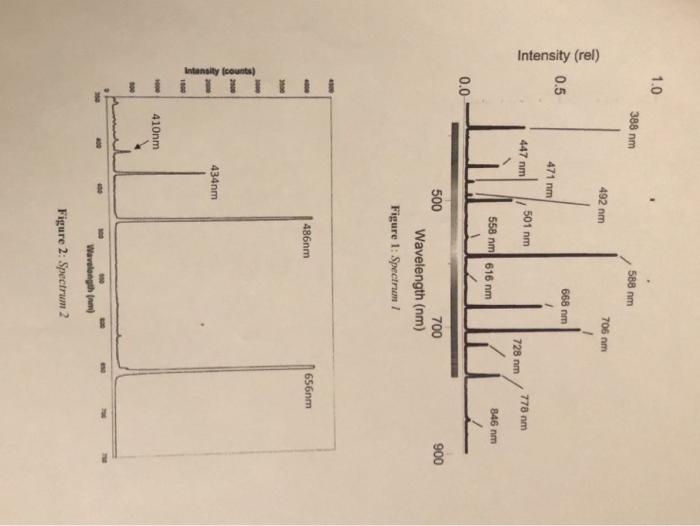# (Solved): just 9 9- One has recorded the spectrum of the hydrogen atom (see attached figures ). From the two ...just 99- One has recorded the spectrum of the hydrogen atom (see attached figures ). From the two recorded spectra (spectrumI and spectrum 2), what is the spectrum corresponding to hydrogen atom? Justify your response. Figure 1: Spectrum I Figure 2: Spectrum 2 7. Consider a transition between two energy levels $$E_{n}$$ and $$E_{n \prime}$$, where $$n^{\prime}>n$$. Compute the expression of this transition energy (denoted $$\left.\Delta E_{n \rightarrow n}\right)$$. Deduce the wavelength $$\left(\lambda_{n \rightarrow n}\right)$$. 8- Complete the following table. 9- One has recorded the spectrum of the hydrogen atom (see attached figures). From the two recorded spectra (spectruml and spectrum 2), what is the spectrum corresponding to hydrogen atom? Justify your response. Useful formulas and numerical constants: - $$h=6.624 \times 10^{-34} \mathrm{~J} . s ; \quad c=3 \times 10^{5} \mathrm{~m} / \mathrm{s} ; \quad q=1.602 \times 10^{-19} \mathrm{C} ; \quad \omega=8.85 \times 10^{-12} \mathrm{C}^{2} / \mathrm{Nm}^{2} ; \quad m=9.1 \times 10^{-}$$ $${ }^{31} \mathrm{Kg}: M=1.7 \times 10^{-27} \mathrm{Kg}$$. - $$\int_{0}^{+\infty} x^{n} e^{-\alpha x} d x=\frac{n !}{\alpha^{n+1}}$$.

We have an Answer from Expert